Courses

# Test: Thermodynamics (M)

## 25 Questions MCQ Test Chemistry Class 11 | Test: Thermodynamics (M)

Description
This mock test of Test: Thermodynamics (M) for JEE helps you for every JEE entrance exam. This contains 25 Multiple Choice Questions for JEE Test: Thermodynamics (M) (mcq) to study with solutions a complete question bank. The solved questions answers in this Test: Thermodynamics (M) quiz give you a good mix of easy questions and tough questions. JEE students definitely take this Test: Thermodynamics (M) exercise for a better result in the exam. You can find other Test: Thermodynamics (M) extra questions, long questions & short questions for JEE on EduRev as well by searching above.
QUESTION: 1

### Consider the reaction at 300K H2(9) + Cl2(9)  →  2HCI(g),  ΔH° = — 185 KJ  If 3 mole of HE completely react with 3 mol of CI2 to form CI, AU° of the reaction will be

Solution: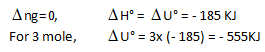QUESTION: 2

### It has been observed that the ratio of the molar heat of vaporisation of liquid and its normal boiling point is approximately same for most of the liquids (called Triton's rule) Which of the following does not follow this rule

Solution:

The correct answer is Option D.

Due to hydrogen bonding in the liquid state there is less entropy than expected and it shows some negative deviation in the Trouton's rule. In vapor state there is more entropy and it shows positive deviation in the rule. Thus for ammonia the Trouton's rule is not applicable.

QUESTION: 3

### A cyclic proces ABCD is shown in PV diagram for an ideal gas.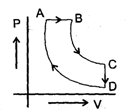Which of the following diagram represents the same process

Solution:

In such type of graph conversions, the best way to work is to make a parameter constant and then derive the results between others.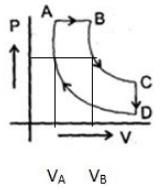Let us make pressure constant. Then we have, VB>VA
Or
(nrTA/P)<(nrTB/P) or TA<TB
In terms of V-T curve, the thing happens as follow:-
A to B (Volume increase from VA to VB. No change in pressure). B to C (temperature is constant), C to D (Volume remains constant) and D to A (Volume decreases but temperature remains constant. However the constant value is less than from B to C). On these all conditions, only graph d stands.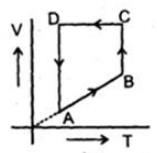QUESTION: 4

The change in free energy accompanied by the isothermal reversible expansion of 1 mol of an ideal gas when it doubles its volume is ΔG1 The change in free energy accompanied by sudden isothermal irreversible doubling volume of 1 mole of the same gas is ΔG2 Ratio of ΔG1 and ΔG2

Solution:

∆G=nR∆T
Therefore, ∆G1/∆G2=1

QUESTION: 5

The following curve represents the variation of Gibbs function 'G' with pressure at constant temperature.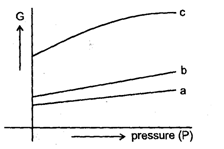Correct match of given plots with the physical state of a substance is

Solution:

Slope: positive
V cannot be negative
Effect of pressure on solids and liquids is negligible. So b must be liquid and a be solid
For gases, as pressure increase volume decrease and slope also decreases and c is graph with decreasing slope so c must be gas

QUESTION: 6

Which of the following diagram correctly represents the variation of internal energy (U) of gas under expansion at constant temperature.

Solution:
QUESTION: 7

Which correctly represents the entropy (s) of an isolated system during a process

Solution:

The second law of thermodynamics states that the entropy of an isolated system never decreases, because isolated systems always evolve toward thermodynamic equilibrium, a state with maximum entropy.
Also, entropy cannot be negative. It is zero for pure crystalline substances only at absolute zero. So, for a general case, we can choose C

QUESTION: 8

Which of the following represents positive work i.e. work of compression

Solution:
QUESTION: 9

For a perfectly crystalline solid Cp m = aT3, where a is constant. If a is 0.42 J/K-mol at 10 K, molar entropy at 10 K is

Solution: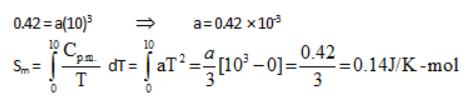QUESTION: 10

One mole of an ideal monoatomic gas expands isothermally against constant external pressure of 1 atm from initial volume of 1L to a state where its final pressure becomes equal to external pressure. If initial temperature of gas is 300 K then total entropy change of system in the above process is :
[R = 0.082 L atm mol-1 K-1 = 8.3 J mo1-1K-1].

Solution:

∆S = nR ln(Vf/Vi) = R ln(Pi/Pf) = R×ln(300R/1L×1 atm) = R ln(24.6)

QUESTION: 11

At 1000 K water vapour at 1 atm. has been found to be dissociated into H2 and 02 to the extent of 3 x 10-6 %.Calculate the free energy decrease of the system, assuming ideal behaviour.

Solution: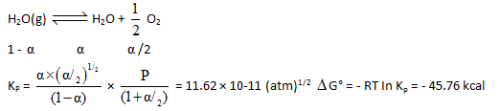QUESTION: 12

In the reaction COCl2(g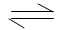C0(g) + CI2(g) at 550°, when the initial pressure of CO & Cl2 are 250 and 280 mm of Hg respectively. The equilibrium pressure is found to be 380 mm of Hg. Calculate the degree of dissociation of COC12 at 1 atm. What will be the extent of dissociation, when N2 at a pressure of 0.4 atm is present and the total pressure is 1 atm.

Solution: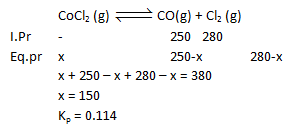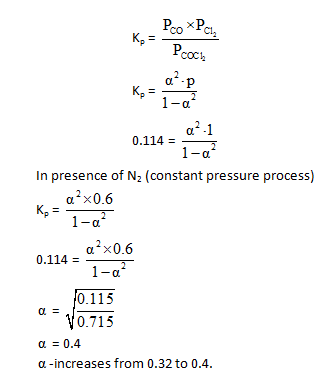QUESTION: 13

When 1 mole of an ideal gas at 20 atm pressure and 15 L volume expands such that the final pressure becomes 10 atm and the final volume become 60 L. Calculate entropy change for the process (Cpm = 30.96 J mole-1 K-1)

Solution:

From ideal gas equation, P1V1/T1 = P2V2/T2
Given that T2/T1 = 6/3 = 2
And V1 = 15 L and V2 = 60 L
P1/P2 = 2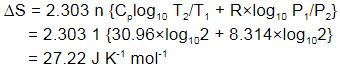QUESTION: 14

An ideal gas is taken from the same initial pressure P1 to the same final pressure P2 by three different processes. If it is known that point 1 corresponds to a reversible adiabatic and point 2 corresponds to a single stage adiabatic the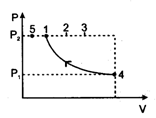Solution:

Since work done on the system, is maximum in single stage compression hence all other adiabatic points will fall between 1 and 2.

QUESTION: 15

Which of the following graphs best illustrates the variation of entropy of a substance with temp.

Solution:

At phase transitions, entropy increases at constant temperature and it is ero at absolute zero.

QUESTION: 16

During winters, moisture condenses in the form of dew and can be seen on plant leaves and grass. The entropy of the system in such cases decreases as liquids possess lesser disorder as compared to gases. With reference to the second law, which statement is correct, for the above process ?

Solution:

As dew formation is spontaneous process therefore entropy or randomness of the universe will increase. As randomeness of system has decreased but randomness of the surrounding will increase larger so that change is positive

QUESTION: 17

A copper block of mass `m' at temperature 'T1' is kept in the open atmosphere at temperature `T2' where T2 > T1 . The variation of entropy of the copper block with time is best illustrated by

Solution:

There is some finite value of entropy at t = 0. It increases constantly till the temperature of the copper block and surroundings is equal. This will be the equilibrium situation hence further change in entropy is restriticted ΔS = 0 at equilibrium.

QUESTION: 18

For which process will AHD° and AG° be expected to be most similar.

Solution:

All product and reactants are in solid state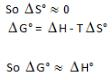QUESTION: 19

Statement-1 : Due to adiabatic free expansion temperature of real gas may increase

Statement-2 : In adiabatic free expansion, temperature is always constant irrespective of real or ideal gas

Solution:

For ideal gas, change in internal energy=ΔU∝ΔT
However for real gases, ΔU=f(V,T)
Thus T may change in this case.
So, statement I is true while statement II is false.

QUESTION: 20

Statement-1 : Under free expansion ,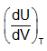is + ve when attractive forces are dominant between gas dB molecules [U, V, T respresent internal energy, volume and temperature of gas respectively]

Statement-2 Internal energy is a state function.

Solution:

When attractive forces are dominant on volume increase particles will move away against attraction force for this system requires extra energy at constant temperature, hence internal energy will increases hence (dV/dU)T is +ve.

QUESTION: 21

The enthalpy of fusion of water is 1.435 kcal/mol. The molar entropy change for the melting of ice at 0°C is

Solution: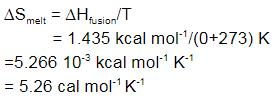QUESTION: 22

Statement-1 : 'Diamonds are forever is generally quoted for diamond as rate of conversion of diamond to graphite at room condition is nearly zero.

Statement-2 : At room condition, conversion of diamond into graphite is spontaneons.

Solution:

Thermodynamically it is favourable for diamond to turn into graphite, but it do not normally happen because there is high energy of activation for the process. So, option b is correct.

QUESTION: 23

Statement-1 : At low temperature, ΔH is the dominant factor for spontaneity of a reaction.
Statement-2 : The opposing factor TΔS remains very small, at low temperature.

Solution:

We know that ∆G = ∆H - T∆S
For low temperature, ∆H is the dominating factor as other other term won't remain significant. So both statements are correct with S II the correct explanation of SI.

QUESTION: 24

Statement-1 : The amount of work done in the isothermal expansion is greater than work done in the adiabatic system for same final volume.

Statement-2 : In the adiabatic expansion of a gas temperature and pressure both decrease due to decrease in internal energy of the system.

Solution:

∵ P & T both decreases hence pressure is less than the pressure of isothermal process.
∴ Work done in reversible adiabatic is less.
Hence, the correct answer is Option A.

QUESTION: 25

Statement-1 : The heat absorbed during isothermal expansion of an ideal gas against vacuum is zero.

Statemept-2 : In isothermal process, change in internal energy is always zero, irrespective of ideal or real gas.

Solution:

The heat absorbed during the isothermal expansion of an ideal gas against vacuum is zero.
During isothermal change, the temperature remains constant.
During expansion in vacuum, the work done is zero. Hence, there is no change in the internal energy.
Statement-2 is true for ideal gas only.